Control Created Edited

# Pushover Global Control

## Function

• Pushover analysis is one of the most commonly used methods for effectively evaluating the post-yield behavior and limit states of a structure in nonlinear static analysis.
• In the evaluation of structural capacity using Pushover analysis, specific analysis conditions are specified.

1. Initial Load Condition : Input the initial gravity load, such as the self-weight of the structure, which is continuously acting on the structure.

2. Analysis Convergence Condition : Input the convergence condition for each step in the nonlinear analysis.

3. Stiffness Reduction Factor : Input the post-yield nonlinear behavior characteristics of the member.

4. Reference Location for Distributed Elements : Select the cross-section location to be referenced for calculating the yield strength of the elements assigned with distributed hinges.

## Call

From main menu, select [Poshover] tab > [Global Control] group >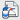[Pushover Global Control]

## Input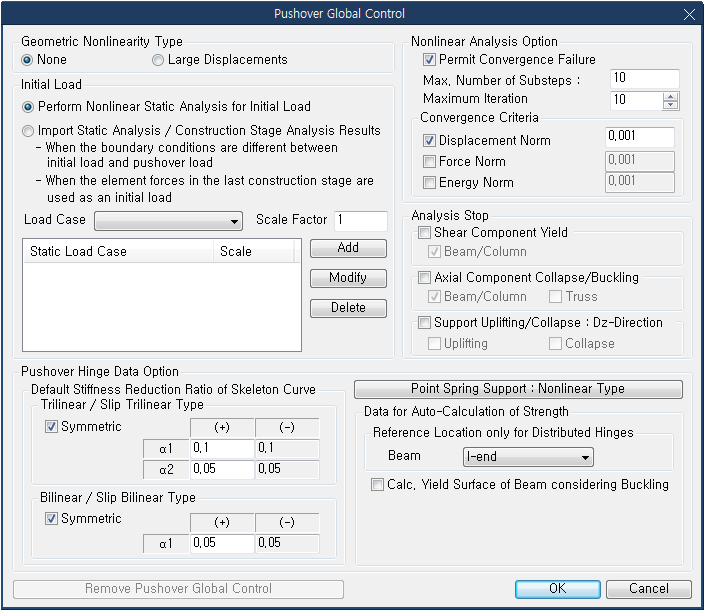Pushover Global Control dialog box

### Geometry Nonlinearity Type

Select Large Displacement to consider the geometric nonlinear effect due to large displacement in Pushover Analysis.

Perform Nonlinear Static Analysis for Initial Load: This is a common approach where the analysis is performed considering the initial load. The structure is subjected to gravity loads, and a nonlinear analysis is carried out to evaluate the accurate capacity curve of the structure.

Perform Nonlinear Static Analysis for Initial Load : This is a common approach where the analysis is performed considering the initial load.

Import Static Analysis / Construction Stage Analysis Results

1. When the boundary conditions are different between the initial load and the pushover load.

2. To use the result from the final construction stage as the initial load.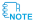When using the result from the final construction stage as the initial load for the pushover analysis, the member forces from the construction stage analysis will be imported and nonlinear analysis will not be performed for the initial load.
For the following cases, nonlinear analysis will not be performed for the initial load but the result from the static analysis will be imported for the pushover analysis.
- When the boundary conditions/section stiffness scale factors are different between the initial load and the pushover load
- When using the member forces from the final construction stage as the initial load for the pushover analysis

Load Case : Select the load cases, which are to be defined as the initial load for pushover analysis, among the load cases applied to static analysis.

Scale Factor : Enter the magnification/reduction factors to be applied to each selected load case for the initial load.

When you click the Add button, the selected load condition will be added to the list. To modify the specified load condition, click the Modify button. To delete it, click the Delete button.The member forces caused by the initial load (in general, the gravity load) are added to the resulting member forces due to pushover analysis. However, the displacements caused by the initial load are not considered in the pushover analysis.
In the case of considering axial force variation (PMM Type), the initial load needs to be defined.If the following temperature loads are entered as an Initial Load, pushover analysis cannot be performed.
1. Beam Section Temperature
3. System Temperature
4. Nodal Temperature
5. Element TemperatureIf P-M interaction is considered in the hinge properties, it is recommended to apply the initial load.In order to check the results due to initial load in the pushover analysis, a linear static analysis for the load cases which are defined as initial load should be performed.

### Nonlinear Analysis Option

Enter the settings for each step applied during the analysis, including maximum iteration count and convergence criteria.

Permit Convergence Failure : This feature automatically divides the step interval and performs the analysis when convergence is not achieved in each step. If convergence is not achieved within the maximum iteration count, the analysis is performed by automatically dividing the step into the specified number of substeps.

Check On : If convergence is not achieved, the analysis proceeds to the next step (Note: The unbalanced forces are considered in the next step's load).

Check Off : If convergence is not achieved, a message is displayed, and the analysis is terminated.

Max. Number of Substeps : Input the maximum number of substeps.The entered number of steps here is applied to all Pushover load conditions.

Maximum Iteration : Input the maximum number of iterations performed to satisfy the equilibrium condition for each incremental step concerning load and displacement.Unbalanced Forces and Convergence Calculation:

Unbalanced Forces and Convergence Calculation:

In each incremental step of nonlinear time history analysis, unbalanced forces (residual forces) occur due to changes in stiffness and member forces. To resolve the unbalanced forces in each step, iterative analysis is performed using the Newton-Raphson method.

1. When performing convergence calculations - The analysis is iterated until the convergence condition is satisfied according to the Newton-Raphson method. During this process, the unbalanced forces become negligible. If the convergence condition is not met and the maximum iteration count is reached, the remaining unbalanced forces are treated as external loads for the next increment.

2. When not performing convergence calculations (setting the maximum iteration count to 1) - The unbalanced forces are treated as external loads for the next increment.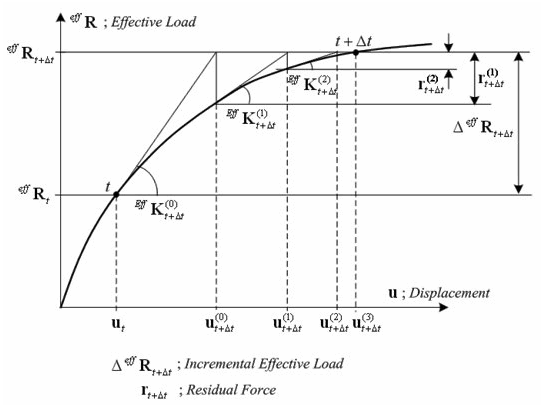Convergence Criteria : Enter the tolerance for determining convergence. If the calculated error during the iterative analysis falls within the specified tolerance, the analysis for that incremental step is terminated within the maximum iteration count, and the analysis proceeds to the next step.Convergence Conditions:

Convergence Conditions:

Even when unbalanced forces are resolved through iterative analysis, achieving complete convergence to zero unbalanced forces is numerically impossible. Therefore, a convergence criterion is set to determine when the unbalanced forces have converged to a certain level, and to proceed to the next increment.

In iterative analysis, there are three criteria (Norms) used to determine convergence: displacement, load, and energy. You can select one or multiple Norms to be considered for convergence determination. The definitions of each Norm are as follows:

Displacement Norm Force Norm Energy Norm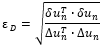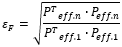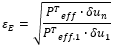여기서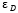: Displacement Norm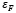: Load Norm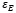: Energy Norm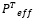: Effective Load Vector at the nth Iteration Step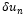: Displacement Increment Vector at the nth Iteration Step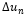: Accumulated Displacement Increment Vector from nth Iterations

Setting Convergence Criteria
It is common to select only the Displacement Norm as the convergence criteria for the analysis. However, even when considering only the Displacement Norm, there can be cases where the residual forces remain significant enough to cause divergence in the analysis. In such cases, additional criteria such as Load and Energy can be selected to perform the analysis.

Considerations when Setting Convergence Criteria

1. When multiple Norms are selected as convergence criteria, the number of iterations required for convergence increases compared to selecting a single criterion.

2. It can be challenging to satisfy the convergence condition when the Energy Norm is chosen.

3. Even if the maximum number of iterations is reached and the convergence criteria are not met, the remaining residual forces are added to the external forces of the next increment. Therefore, if convergence is achieved in the current increment, the overall analysis results are not significantly affected, even if convergence was not achieved in the previous increment.

### Default Stiffness Reduction Ratio of Skeleton Curve

This parameter defines the post-yield behavior of the element.

If the nonlinear hinge skeleton curve is nonlinear/bilinear (or if a slip curve is used), the stiffness reduction ratios for the first and second yield are specified.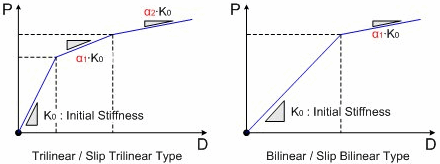Trilinear / Slip Trilinear Type : Enter the stiffness reduction ratios for the first and second yield when defining a trilinear curve.

α1：Stiffness reduction ratio after the first yield (α1 ≤ 1.0)

α2：Stiffness reduction ratio after the second yield (α2 ≤ α1 ≤ 1.0)

Bilinear / Slip Bilinear Type : Enter the stiffness reduction ratio after the first yield when defining a bilinear curve.

α1：Stiffness reduction ratio after the yield (α1 ≤ 1.0)

### Point Spring Support & Elastic Link : Nonlinear Type

For Pushover analysis, you can include the nonlinear properties of Point Spring Supports and Elastic Links.

Point Spring Support : Comp.-only, Tens.-only, Multilinear Type

Elastic Link: Comp.-only, Tens.-only, Multilinear Type

### Data for Auto-Calculation of Strength

When performing Pushover analysis using distributed hinges, specify the section location for calculating the yield strength of the element.

Reference Location only for Distributed Hinges : Select the location of the element section (i-end, j-end, middle) for calculating the yield strength.

Calc. Yield Surface of Beam considering Buckling : Consider buckling when calculating the yield plane of the beam element.

Remove Puchover Global Control : Deletes the information entered in the Pushover analysis conditions, and in such cases, Pushover analysis will not be performed.

0